Loop

(diff) ← Older revision | Latest revision (diff) | Newer revision → (diff)

A quasi-group with an identity, that is, with an elementsuch thatfor every elementof the quasi-group. The significance of loops in the theory of quasi-groups is determined by the following theorem: Any quasi-group is isotopic (see Isotopy) to a loop. Therefore, one of the main problems in the theory of quasi-groups is to describe the loops to which the quasi-groups of a given class are isotopic.

With every loop there are associated three kernels. The setof elements of a loopis called the left kernel. Similarly one defines the middle and right kernels. They always exist in a loop. Their intersection is called the kernel of the loop. Every kernel is an associative subloop, that is, a subgroup of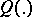. Corresponding kernels of isotopic loops are isomorphic. There are loops with any preassigned kernels. A loopisotopic to a groupis itself a group and is isomorphic to the group(Albert's theorem). In particular, isotopic groups are isomorphic. Some other classes of loops also have this property, for example free loops. A loopis called a-loop if any loop isotopic tois isomorphic to it.

Many concepts and results of group theory can be extended to loops. However, some of the usual properties of a group need not hold for loops. Thus, in finite loops Lagrange's theorem (that the order of a subgroup divides the order of the group) does not hold, generally speaking. If, nevertheless, Lagrange's theorem does hold for a loop, then the loop is said to be Lagrangian. If every subloop of a loopis Lagrangian, one says thathas the property. A necessary and sufficient condition for a loopto have the property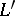is the following:must have a normal chain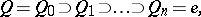where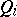is a normal subloop in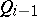, such thathas the propertyfor all.

The loops that have been most studied and are closest to groups are Moufang loops (cf. Moufang loop). The main theorem about them (Moufang's theorem) is: If three elementsof such a loop are connected by the associative law, that is, if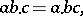then they generate an associative subloop, that is, a group. In particular, any Moufang loop is di-associative, that is, any two elements of it generate an associative subloop. The property of being a Moufang loop is universal, that is, it is invariant under isotopy: Any loop isotopic to a Moufang loop is itself a Moufang loop.

One of the most general classes of loops is the class of IP-loops, or loops with the invertibility property. They are defined by the identitiesHere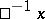andare, respectively, the left and right inverse elements of. Any Moufang loop is an IP-loop. In an IP-loop all kernels coincide. The kernel of a Moufang loop is a normal characteristic subloop. The property of being an IP-loop is not universal. Moreover, if any isotope of an IP-loopis an IP-loop, thenis a Moufang loop.

More general than the class of IP-loops is the class of WIP-loops, or loops with the weak invertibility property. They are defined by the identity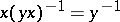. This identity is universal if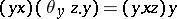for all, whereis an automorphism. In this case the kernel of the WIP-loop is normal and the quotient loop with respect to the kernel is a Moufang loop. A special case of WIP-loops are the CI-loops, or cross-invertible loops, defined by the identity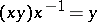.

A generalization of Moufang loops are the (left) Bol loops, in which the identity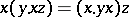holds. They are invariant under isotopy and are mono-associative, that is, every element of such a loop generates an associative subloop.

An important concept in the theory of quasi-groups and loops is the concept of a pseudo-automorphism. A permutationof a loopis called a left pseudo-automorphism if there is an element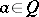such that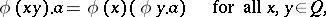and is called a right pseudo-automorphism if there is an elementsuch that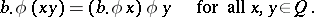Ifis both a left and a right pseudo-automorphism, thenis called a pseudo-automorphism, and the elementsandare called the left and right companions, respectively. For loops an automorphism is a special case of a pseudo-automorphism. Every pseudo-automorphism of an IP-loop induces an automorphism in its kernel, and in a commutative Moufang loop any pseudo-automorphism is an automorphism.

In the theory of loops a significant role is played by inner permutations. A permutationof the associated groupof a loopwith an identityis said to be inner if. The setof all inner permutations is a subgroup ofand is called the group of inner permutations. The groupis generated by permutations of three types: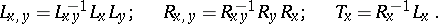By means of inner permutations one can define-loops as loops for which all inner permutations are automorphisms. If an-loop is also an IP-loop, then it is di-associative. Commutative di-associative-loops are Moufang loops. For commutative Moufang loops inner permutations are automorphisms.

Some definitions of group theory carry over to loops. Thus, a loop is said to be Hamiltonian if any subgroup of it is normal. Abelian groups are also regarded as Hamiltonian loops. Mono-associative Hamiltonian loops with elements of finite order are direct products of Hamiltonian-loops (a-loop is defined in the same way as a-group). Di-associative Hamiltonian loops are either Abelian groups or direct products: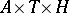, whereis an Abelian group whose elements have odd order,is an Abelian group of exponent 2 andis a non-commutative loop satisfying additional conditions.

A loopis said to be totally (partially) ordered ifis a totally (partially) ordered set (with respect to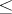) and if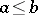impliesand conversely. If the centre of a totally ordered loophas finite index, thenis centrally nilpotent. Lattice-ordered loops with the minimum condition for elements are free Abelian groups.

Loops have also been studied by means of associated groups. It has been proved, for example, that there is a one-to-one correspondence between the normal subloops of a loop and the normal subgroups of the corresponding associated group.

For references see Quasi-group.

How to Cite This Entry:
Loop. Encyclopedia of Mathematics. URL: http://encyclopediaofmath.org/index.php?title=Loop&oldid=15795
This article was adapted from an original article by V.D. Belousov (originator), which appeared in Encyclopedia of Mathematics - ISBN 1402006098. See original article